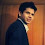# SAS : Calculate AUC of Validation Data

This tutorial explains how to calculate area under curve (AUC) of validation sample. The AUC of validation sample is calculated by applying coefficients (estimates) derived from training sample to validation sample. This process is called Scoring. The detailed explanation is listed below -

Steps of calculating AUC of validation data

1. Split data into two parts - 70% Training and 30% Validation. It can be 60/40 or 80/20.

2. Run logistic regression model on training sample.

3. Note coefficients (estimates) of significant variables coming in the model run in Step 2.

4. Apply the following equation to calculate predictive probability in the validation sampleLogistic Regression Equation
In this case, b0 is intercept and b1...bk - coefficients derived from training sample (Step2)

5. Calculate Area under Curve (AUC) considering probability scores derived in Step 4.

Method I : PROC LOGISTIC to calculate AUC of Validation
Proc Logistic Data = training outest=coeff descending;
class rank / param = ref;
Model admit = gre gpa rank / selection = stepwise slstay=0.15 slentry=0.15 stb;
Run;
Proc Logistic Data = validation inest=coeff descending;
class rank / param = ref;
Model admit = gpa rank / MAXITER=0;
Run;
The OUTEST= option in the PROC LOGISTIC stores final estimates in the SAS dataset. In this case, it is stored on the dataset named COEFF. We have run stepwise regression which drops an insignificant variable named GRE.

The INEST= option in the PROC LOGISTIC uses the final parameter estimates calculated from training dataset.

The MAXITER= option in the MODEL statement specifies the maximum number of iterations to perform. The combination of DATA=validation data, INEST=final estimates from training data, and MAXITER=0 causes PROC LOGISTIC to score, not refit, the validation data.

Important Points
1. Use only significant variables from the training data in MODEL Statement of PROC LOGISTIC DATA = VALIDATION. In the above example, GRE variable was not included.
2. Parameter Estimates (Coefficients) would remain same produced by both PROC LOGISTIC programs as we are scoring in second PROC LOGISTIC program, not building the model.
3. Ignore warning 'Convergence was not attained in 0 iteration' when running second PROC LOGISTIC statement.AUC - Validation

The c-statistics is AUC (Area under Curve). In this case, it is 0.607.

Method II : PROC NPAR1WAY

In this method, we are using Wilcoxon method to calculate AUC of validation sample. First, we are scoring using SCORE statement with VALIDATION sample.
Proc Logistic Data = training descending;
class rank / param = ref;
Model admit = gre gpa rank / selection = stepwise slstay=0.15 slentry=0.15 stb;
score data=validation out = valpred;
Run;

ods select none;
ods output WilcoxonScores=WilcoxonScore;
proc npar1way wilcoxon data= valpred ;
var  p_1;
run;
ods select all;

data AUC;
set WilcoxonScore end=eof;
retain v1 v2 1;
if _n_=1 then v1=abs(ExpectedSum - SumOfScores);
v2=N*v2;
if eof then do;
d=v1/v2;
Gini=d * 2;    AUC=d+0.5;
put AUC=  GINI=;
keep AUC Gini;
output;
end;
run;
The above program returns AUC score of 0.6062.
Related Posts
ShareDeepanshu founded ListenData with a simple objective - Make analytics easy to understand and follow. He has over 10 years of experience in data science. During his tenure, he worked with global clients in various domains like Banking, Insurance, Private Equity, Telecom and HR.

7 Responses to "SAS : Calculate AUC of Validation Data"
1.Hi Deepanshu,

Very interesting this post and the previous of interactions in logistic regression.

I am not an expert in models and I have doubts abouy AUC calculation. As far as
I know AUC is area under ROC curve. But I don't understand how the points of ROC
curve are calculated. When I validate a model I only have one point, that is a value for true positive rate and a value for false positive rate (sensitivity and specificiry..), I don't know how to get several points.....ti build a curve...

Perhaps is a newby doubt....

1.You can use the following program to generate ROC curve. The OUTROC= option stores sensitivity and (1-specificity) of various points in SAS dataset.

proc logistic data=training noprint;
model y = x / outroc=ROCData;
run;

symbol1 v=dot i=join;
proc gplot data=ROCData;
plot _sensit_*_1mspec_;
run;quit;

2.Another question: ¿whay is the meaning of _PROB_in the outpit dataset?, ¿what do represent?. Thanks

2.Hi Deepsanshu, may I know where can I get the sample data "training"? Appreciate your help

3.HI Deepanshu.

Proc Logistic Data = develop_valid inest=coeff ;
Model ins(event = '1')= DDA DDABal Checks Sav SavBal CD MMBal CC /MAXITER=0; *these are the lsit of variables which are significant.*

Now to find the predicted probability in validation dataset i am using parameter values of training dataset to find P_1.

Proc Logistic Data = develop_train_vif outest=coeff ;
Model ins(event = '1')= DDA DDABal Checks Sav SavBal CD MMBal CC ;
Run;

Proc Logistic Data = develop_valid inest=coeff ;
Model ins(event = '1')= DDA DDABal Checks Sav SavBal CD MMBal CC /MAXITER=0;
score data=develop_train_vif out=validation_probabilities;
Run;

is the above code coorect toscore values in validation dataset.?
plz revert.

Run;

4.5.Hi, can you provide the code to create gAUC

Next → ← Prev
Looks like you are using an ad blocker!

To continue reading you need to turnoff adblocker and refresh the page. We rely on advertising to help fund our site. Please whitelist us if you enjoy our content.